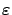Next: Preliminaries Up: -MDPs: Learning in Varying Previous: -MDPs: Learning in Varying

# Introduction

In a common formulation of the reinforcement learning (RL) problem an agent improves its behavior by observing the outcomes of its own interactions with the environment. In the 1980's, Markovian decision problems (MDPs) were proposed as a model for the analysis of RL (for an overview, see [Sutton and Barto(1998)] and references therein), and since then a mathematically well-founded theory has been constructed for a large class of RL algorithms [Watkins(1989),Watkins and Dayan(1992),Tsitsiklis(1994),Gullapalli and Barto(1994),Jaakkola et al.(1994)]. RL algorithms typically consider stationary environments. Quasi-stationary environments are approached by continually adapting the agent. However, changes in the environment may be very fast and could prohibit optimizations for methods that assume a stationary environment.

To provide a principled framework for RL in fast changing environments, we introduce a model called-MDP, a generalization of-stationary MDP [Kalmár et al.(1998)]. In this novel MDP concept the environment is allowed to change over time, provided that the accumulated changes remain bounded. In particular, transition probabilities may vary as a function of time. The only requirement is that the change is finite but small (it is bounded by a small number). We cannot expect to find an optimal policy; it may not even exist for this case. Nevertheless, we prove the following important result using the generalized MDP framework of [Szepesvári and Littman(1996)]: if a reinforcement learning algorithm that approximates the optimal value function converges to the optimal value function in the MDP model, then in the corresponding-MDP the asymptotic distance of the optimal value function and its approximation is bounded, and the bound is proportional tounder the same conditions. The main result of our paper is as follows: If an RL algorithm can learn an optimal policy in an MDP, then it is capable of learning a near-optimal policy in an-MDP as well.

We shall illustrate-MDP in conjunction with a novel reinforcement learning algorithm called event-learning [Lorincz et al.(2002)]. In typical RL formulations, the agent learns an optimal policy that prescribes the optimal action for any given state. This kind of policy has much the same advantages and drawbacks as conditioned reflexes: it can solve difficult tasks, but it may be sensitive to minor changes in the environment. Furthermore, new parameter settings for the same problem may involve having to restart learning from the beginning. In event-learning, the policy selects desired successor states instead of selecting actions. Consequently, not state-action values but the values of state-state pairs (events) are learned. The task of bringing the agent to the desired successor state is passed to a lower-level controller. It has been shown elsewhere [Szita et al.(2002)] that event-learning with a particular non-Markovian controller, the SDS controller [Szepesvári and Lorincz(1997)], belongs to the-MDP problem family under certain conditions. Convergence of learning of-MDP s is studied via computer simulations on the two-link pendulum problem. These simulations intend to demonstrate that-MDPs can be applied, e.g., to various models with uncertain and/or noisy state description.

We will argue that-MDPs can be related to RL methods making use of control ideas [Doya(1996),Doya(2000),ten Hagen(2001)] and to module-based RL [Maes(1992),Mahadevan and Connell(1992),Mataric(1997),Kalmár et al.(1998)].

The article is organized as follows. Section 2 provides an overview of MDPs and generalized MDPs. We introduce the concept of generalized-MDPs and the appropriate generalized Q-learning in Section 3 and prove the main `convergence' theorem for generalized-MDPs. In Section 4 an overview of event-learning is provided. Section 5 contains illustrations of the-MDP model within the event-learning framework using the two-link pendulum problem. Conclusions are drawn in Section 6. The paper concludes with an appendix providing mathematical details on-MDPs, including the proof that event-learning algorithm augmented with an adapting non-Markovian controller can form an-MDP problem.Next: Preliminaries Up: -MDPs: Learning in Varying Previous: -MDPs: Learning in Varying